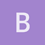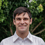This is an archived static version of the original phylobabble.org discussion site.

# Counting topologies with complex constraintsjembrown

Does anyone know of a solution to count all possible topologies consistent with a complex set of constraints (including hard positive, hard negative, and partial positive)? Ideally, I’m interested in existing software solutions, but theory leads are welcome too.ematsen

Are your constraints equivalent to splits, i.e. these taxa form an unrooted clade?

Could you please clarify a little what you mean by hard vs partial?jembrown

Apologies for the ambiguity. Yes, the constraints are equivalent to splits. Regarding hard vs. partial, I was using the terminology employed by MrBayes. Hard constraints are strict and define a split for all taxa. Partial (or backbone) constraints are defined for a subset of taxa, with the remainder allowed to occur on either side of the split.mathmomike

A couple of further questions:

1. are you interested in counting (i) all phylogenetic trees, or (ii) just all binary ones, or (ii) all minimally-refined trees, subject to the constraints?

Also, are you working with rooted trees (and so clusters rather than splits) or unrooted trees?jembrown

We’re interested in counting all binary, unrooted trees.mathmomike

Hi, ok this might help.

Let S= \{\sigma_1,..., \sigma_k\} be a set of splits you want to be in your (binary) trees, and let N_S be the number of binary trees with these splits. Note that N_S=0 unless the splits are pairwise compatible, and if they are, then they form a unique minimally-resolved tree T_S (generally not binary). Then there is a simple formula for N_S, namely

N_S = \prod_{v \in IV(T_S)} b(deg(v))$where IV(T_S) is the set of interior vertices of$T_S, deg(v) is the degree of vertex v in T_S, and b(k) = (2k-5)!! is the number of unrooted binary tree in k leaves = 1x 3 x … x(2k-5)

For example if S= {123|45678, 1234|5678} then T_S (good idea to draw it) has interior vertices of degrees 3,4 and 5 and so N_S = b(3)xb(4)xb(5) = 1x(1x3)x (1x3x5) = 45.

Now suppose that you also want to exclude certain splits - so you have a second set \Sigma’ = {\sigma’1,…, \sigma’k}\$ and you want your (binary) tree to also exclude each split in this set. So let N{S,S’} be the number of binary trees that have each split in S and no split in S’. You can now just use the previous result (for N_S) in concert with the “principle of inclusion and exclusion” PIE (see Wikipedia if that is not familiar). For example, in our previous example, if we want to also exclude two splits by taking S'= \{56|....., 78|....\} (where … just means the other taxa) then the PIE (and the theory from the previous paragraph) tells us that this will be: N_S - N(S + 56|…) - N_(S+ 78|…) + N_(S+56|…+ 78|…) = 45 - (1x 3 x 3) - (1x 3 x 3) + (1 x 3 x 1) = 30 (it’s worth drawing the last 3 trees to check the degrees of the vertices).

Finally if you have partial information (e.g. partial splits like 14|67) you want to include - then in general there is not good algorithm since even if all you have is s set of quartet splits Q (like 14|67) and no other full splits at all to insist on or exclude (as above) then even deciding whether or not N_Q=0 is NP-hard.

However if there is some taxon/leaf that is present in all the partial splits, then an algorithm is possible (based on the Aho et al. algorithm, and the counting approach developed by Constantinescu and Sankoff (SUPERB I think it was called)).

Hope that helps. Just get back if you have any questions (here or by email). best, Mike Steeljembrown

Mike, thanks much, this is very helpful. The partial constraints have been giving us the most trouble, although we do have several taxa that are present in all partial splits. Is this the Constantinescu and Sankoff paper that you were referencing?

An efficient algorithm for supertrees. 1995. Journal of Classification. 12:101-112.

This is probably overly hopeful, but do you know of any existing software implementation of this algorithm?

Best, Jeremy Brownmathmomike

Hi Jeremy,

Pleased that helps. yes, that’s the paper I meant … I think Michael Sanderson (Arizona) may have an implementation of that algorithm - you could email him and ask (username is sanderm, domain is email.arizona.edu). best, Mikeematsen

Thanks a bunch, @mathmomike! I’ve just invited Michael Sanderson to phylobabble (and slightly obscured his email address in your post).

It would be great to continue the conversation here for others who are interested in this topic.